How Cheenta works to ensure student success?
Explore the Back-Story

# Positive Integer | PRMO-2017 | Question 1Try this beautiful Positive Integer Problem from Algebra from PRMO 2017, Question 1.

## Positive Integer - PRMO 2017, Question 1

How many positive integers less than 1000 have the property that the sum of the digits of each such number is divisible by 7 and the number itself is divisible by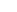•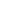•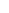•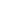### Key Concepts

Algebra

Equation

multiplication

Answer:PRMO-2017, Problem 1

Pre College Mathematics

## Try with Hints

Let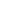be the positive integer less than 1000 and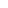be the sum of its digits, then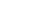and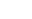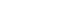therefore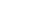Can you now finish the problem ..........

Also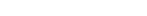therefore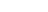Clearly, n must be a 3 digit number Let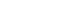be the digits, then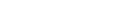where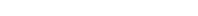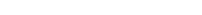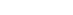Can you finish the problem........

For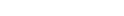the equation (1) has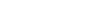solutions
therefore total possible solution of equation (1)

=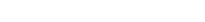## Subscribe to Cheenta at Youtube

Try this beautiful Positive Integer Problem from Algebra from PRMO 2017, Question 1.

## Positive Integer - PRMO 2017, Question 1

How many positive integers less than 1000 have the property that the sum of the digits of each such number is divisible by 7 and the number itself is divisible by•••### Key Concepts

Algebra

Equation

multiplication

Answer:PRMO-2017, Problem 1

Pre College Mathematics

## Try with Hints

Letbe the positive integer less than 1000 andbe the sum of its digits, thenandthereforeCan you now finish the problem ..........

AlsothereforeClearly, n must be a 3 digit number Letbe the digits, thenwhereCan you finish the problem........

Forthe equation (1) hassolutions
therefore total possible solution of equation (1)

=## Subscribe to Cheenta at Youtube

This site uses Akismet to reduce spam. Learn how your comment data is processed.

### Knowledge Partner